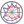Course title
Applied Mathematics
 sasano isaoCourse description
Discrete Fourier transform (DFT) is used for processing sounds and graphics in digital computers. This lecture aims at being able to do Fourier series expansion, which forms the basis for DFT. As an introduction to Fourier series expansion we illustrate the least-square method and the orthogonal function expansion. Fourier series expansion is an instance of the orthogonal function expansion. Understanding Fourier series expansion forms the basis for understanding Fourier transform and DFT, which are topics covered in lectures of signal processing.
Purpose of class
By learning the least-square method, the orthogonal function expansion, and Fourier series expansion, we acquire the basics for processing signals like sounds and images.
Goals and objectives
1. Understanding the least-square method and being able to approximate given sequences of data or functions by linear functions or quadratic functions
2. Understanding orthogonal functions and being able to do the orthogonal function expansion for given functions by some given set of orthogonal functions
3. Understanding Gram-Schmidt orthogonalisation, which is a method (algorithm) for orthogonalising a set of vectors in an inner product space, and being able to construct an orthogonal set of functions from a given set of functions.
4. Being able to do Fourier series expansion, which is an important instance of the orthogonal function expansion.
Language
English
Class schedule

Class schedule HW assignments (Including preparation and review of the class.) Amount of Time Required
1. Introduction and the least-square method (1)
- Approximation of sequences of data in linear functions
Read Section 20.5 of the reference book. 180minutes
2. The least-square method (2)
- Approximation of sequences of data in quadratic functions
Example 2 in Section 20.5 of the reference book 190minutes
3. The least-square method (3)
- Approximation of sequences of data in linear combination of some fixed set of functions
It is not treated in the reference book. 190minutes
4. The least-square method (4)
- Approximation of functions in linear combination of some fixed set of functions
Confer Problem 16 in Section 20.5 of the reference book. 190minutes
5. The least-square method (5) and the orthogonal function expansion(1)
- Approximation of column vectors
- Approximation of functions in linear combination of some fixed set of orthogonal functions
- An orthogonal set of functions --- Legendre polynomials
Read Section 5.7 and 5.8 of the reference book.
Confer Example 2 in Section 5.8 for Legendre polynomials.
Confer Section 7.1 for column vectors.
190minutes
6. The orthogonal function expansion (2)
- An orthogonal set of functions --- Trigonometric functions
- The orthogonal function expansion
Read Section 5.8 of the reference book.
Confer Appendix 3 for formulae about trigonometric functions.
190minutes
7. Mid-term examination and explanation of the answers
- Pencil-and-paper test for checking the understanding of the contents of the lectures from the first to the eighth
(We resume the lecture after the mid-term examination.)
Review the contents of all the lectures until the last one.
Confer Problem 20 in Section 5.7 for Chebyshev polynomials
190minutes
8. The orthogonal function expansion (3)
- An example of the orthogonal function expansion --- Fourier series expansion
- Orthogonal set of functions with a weight function
- An example --- Chebyshev polynomials
Read Section 11.1 for Fourier series expansion. 190minutes
9. The orghogonal function expansion (4)
- Examples --- Hermite polynomials and Laguerre polynomials
Confer Problem 18 in Section 5.8 for Hermite polynomials.
Confer Example 2 in Section 5.8 for Legendre polynomials.
190minutes
10. The orthogonal function expansion (5)
- The orthogonal function expansion in Chebyshev, Hermite, and Laguerre polynomials
- Inner product spaces
- An inner product space --- n-dimensional Euclidean space
Read Section 5.7 and 5.8 of the reference book for the orthogonal function expansion.
Read Section 7.9 for the inner product spaces.
Confer Example 3 in Section 7.9 for the n-dimensional Euclidean space.
190minutes
11. The orthogonal function expansion (6)
- Cauchy&#8211;Schwarz inequality
- Triangle inequality
- Orthonormal basis
Read Section 7.9 for Cauchy-Schwarz inequality and Triangle inequality.
Read Section 5.7 for the definition of orthonormality
Confer Section 7.4 for basis.
190minutes
12. The orthogonal function expansion (7)
- Orthogonal projection
- Orthogonal basis
- Gram-Schmidt orthogonalisation
Read Section 9.2 for projections.
Gram-Schmidt orthogonalisation is not treated in the reference book. Consult some linear algebra textbook.
190minutes
13. The orthogonal function expansion (8)
- Obtaining Legendre polynomials by Gram-Schmidt orthogonalisation
Gram-Schmidt orthogonalisation is not treated in the referece book. Consult some linear algebra textbook. 190minutes
14. Final examination and explanation of the answers
- Paper-and-pencil test for checking the understanding of the contents of the lectures from the first to the fourteenth
Review the contents of all lectures 190minutes
15.
Total. - - 2650minutes
Relationship between 'Goals and Objectives' and 'Course Outcomes'

reports mid-term exam final exam Total.
1. 5% 30% 20% 55%
2. 0% 10% 5% 15%
3. 0% 0% 5% 5%
4. 5% 0% 20% 25%
Total. 10% 40% 50% -
Evaluation method and criteria
Mid-term exam is evaluated on a 40-point scale, final exam a 50-point, and reports a 10-point. When the mid-term exam is M point, the final exam F point, and the repots R point, the overall score is R+M+F*(100-(R+M))/50.
Textbooks and reference materials
A reference book is:
Erwin Kreyszig, Advanced Engineering Mathematics, John Wiley & Sons Inc; 9th International edition, 2006.
The tenth edition was published in 2011 and it may be equally fine.
Note that I do not use this book as a textbook. Note also that this book is thick and covers topics much more than this lecture covers.
Prerequisites
Basic knowledge of linear algebra and analysis
Office hours and How to contact professors for questions
• Friday 13:10-14:50 or any time agreed on by email
Relation to the environment
Non-environment-related course
Regionally-oriented
Non-regionally-oriented course
Development of social and professional independence
• Course that cultivates a basic problem-solving skills
Active-learning course
More than one class is interactive
Last modified : Wed Oct 17 07:11:05 JST 2018